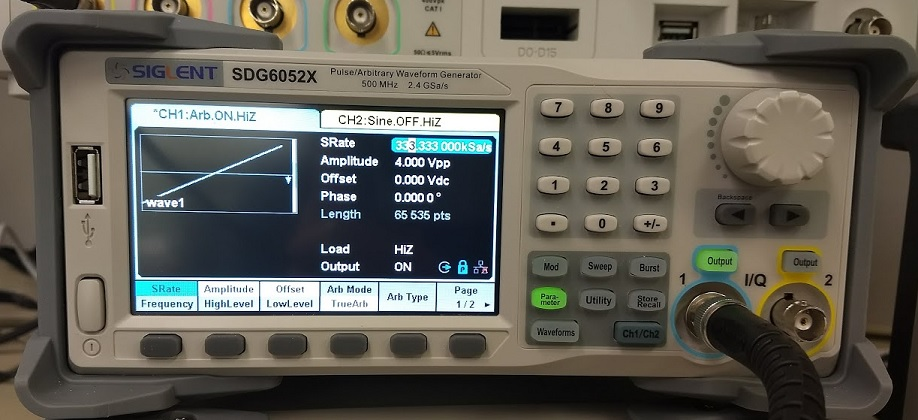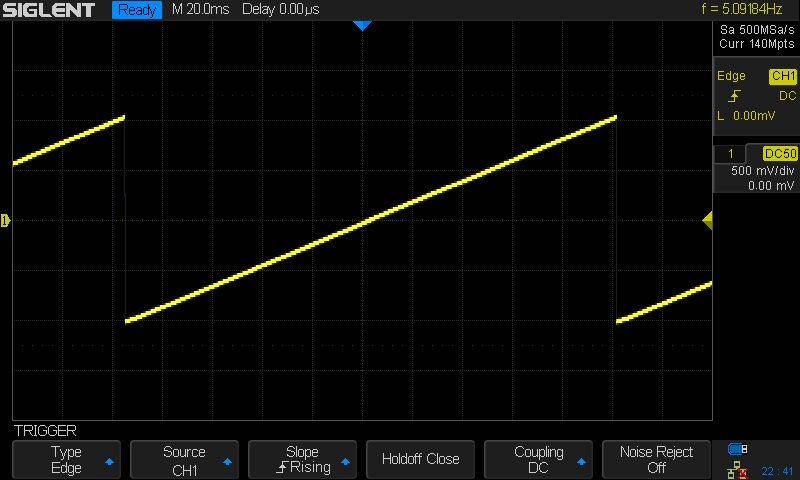# Programming Example: Building an Arb with 16-bit steps (SDG2000X/SDG6000X) using Python

March 7, 2018

The SIGLENT SDG2000X and SDG6000X feature 16-bit voltage step resolution. This provides 65,535 discrete voltage steps that can cover the entire output range (20 Vpp into a High Z load) which can effectively be used to test A/D converters and other measurement systems by sourcing waveforms with very small changes.

In this example, we use Python 2.7 and PyVISA 1.8 to create a ramp waveform that is comprised of steps of the Least Significant Bit (LSB) from point 0 to 65535 on Channel 1.

We also implement the TrueArb function that allows you to specify the sample rate and also ensures that each sample is sourced.

NOTE: You will need to change the instrument ID to match your specific instrument. We also recommend setting the amplitude and other instrument parameters prior to enaling the output of the instrument.

This example can also be altered for use with SDG1032X units by changing the waveform data to match the 14-bit data depth. 14 bits is 0 to 16,384 in decimal notation which is -8192 to 8192 in 2s complement.. in hex this is 0Xe000 (-8192) to 0x2000 (8192).Here is a scope shot of the output:Here is a link to a Zipped version of the .PY file: SiglentSDG16BBitSteps

Here is the text of the program:

```#USB resource of Device
rm = visa.ResourceManager()
device = rm.open_resource('USB0::0xF4EC::0x1101::SDG6XBAQ1R0071::INSTR')

#Little endian, 16-bit 2's complement
#create a waveform

wave_points = []
for pt in range(0x8000, 0xffff, 1):
wave_points.append(pt)
wave_points.append(0xffff)
for pt in range(0x0000, 0x7fff, 1):
wave_points.append(pt)

def create_wave_file():
"""create a file"""
f = open("wave1.bin", "wb")
for a in wave_points:
b = hex(a)
#print 'wave_points: ',a,b
b = b[2:]
len_b = len(b)
if (0 == len_b):
b = '0000'
elif (1 == len_b):
b = '000' + b
elif (2 == len_b):
b = '00' + b
elif (3 == len_b):
b = '0' + b
b = b[2:4] + b[:2] #change big-endian to little-endian
c = binascii.a2b_hex(b) #Hexadecimal integer to ASCii encoded string
f.write(c)
f.close()

def send_wave_data(dev):
"""send wave1.bin to the device"""
f = open("wave1.bin", "rb") #wave1.bin is the waveform to be sent